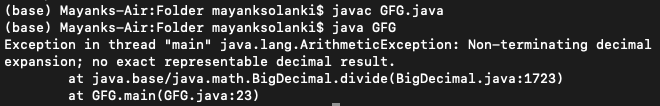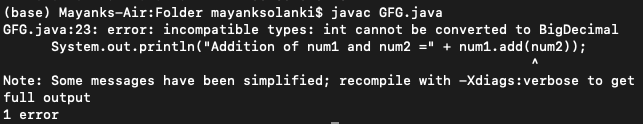# Java Program For Arithmetic Operations Between BigDecimal and Primitive Data Types

The floating-point data types (float and double) are not as accurate to be used in the financial calculations. Therefore, Java offers a separate class “BigDecimal” to perform the operations and avoid the minimal chances of mistakes in calculations. BigDecimal class provides operations on double numbers for arithmetic, scale handling, rounding, comparison, format conversion, and hashing. It can handle very large and very small floating-point numbers with great precision but compensating with the time complexity a bit. BigDecimal provides numerous methods to be implemented upon. In this article, we’ll be discussing the basic arithmetic operations which can be performed on BigDecimal and also performing the same operations between BigDecimal and primitive data types such as int, short, long, etc.

Example 1:

## Java

 `// Java Program to Add, Subtract and Multiply ` `// Two big decimal numbers  `   `// Importing input output classes` `import` `java.io.*;`   `// Importing BigDecimal class from` `// java.math package` `import` `java.math.BigDecimal;`   `// Main class` `class` `GFG {`   `    ``// Main driver method` `    ``public` `static` `void` `main(String[] args)` `    ``{`   `        ``// Declaring two variables of type BigDecimal` `        ``// Custom numbers for illustration` `        ``BigDecimal num1 = ``new` `BigDecimal(``"15.3514628768"``);` `        ``BigDecimal num2 = ``new` `BigDecimal(``"45.37844"``);`   `        ``// Mathematic operation over two numbers`   `        ``// Addition using add() method` `        ``System.out.println(``"Addition of num1 and num2 = "` `                           ``+ (num1.add(num2)));`   `        ``// Subtraction using subtract() method` `        ``System.out.println(``"Subtraction of num1 and num2 = "` `                           ``+ (num1.subtract(num2)));`   `        ``// Multiplication using multiply() method` `        ``System.out.println(` `            ``"Multiplication of num1 and num2 = "` `            ``+ (num1.multiply(num2)));` `    ``}` `}`

Output

```Addition of num1 and num2 = 60.7299028768
Subtraction of num1 and num2 = -30.0269771232
Multiplication of num1 and num2 = 696.625437067096192```

Output Explanation:

Note that the accuracy of the operations is very high. Now performing division on the same set of numbers in the below example as follows:

Example 2:

## Java

 `// Java Program to show exception thrown if blunt division` `// of two big decimal numbers is carried on `   `// Importing input output classes ` `import` `java.io.*;` `// Importing BigDecimal class from` `// java.math package ` `import` `java.math.BigDecimal;`   `// Main class` `class` `GFG {` `  `  `    ``// Main driver method ` `    ``public` `static` `void` `main (String[] args) {` `      `  `        ``// Creating an object of bigDecimal class and` `        ``// initializing the big decimal values` `        ``// Custom entry  ` `        ``BigDecimal num1 = ``new` `BigDecimal(``"15.3514628768"``);` `        ``BigDecimal num2 = ``new` `BigDecimal(``"45.37844"``);` `      `  `        ``// Division over two numbers ` `        ``// using divide() method and printing the resultant number` `        ``System.out.println(``"Division of num1 and num2 = "` `+ (num2.divide(num1)));` `    ``}` `}`

Output:Output explanation:

This error occurred because the division of the two numbers was non-terminating, and we know that BigDecimal is introduced to provide the maximum accuracy. Hence, it produces an error. We will be rectifying the same in the next code where we will be dividing the same numbers, but now the data type is double, and it should not produce any error and gives some answers.

Example 3:

## Java

 `// Java Program to show division of two big decimal numbers`   `// Importing input output classes` `import` `java.io.*;`   `// Main class` `class` `GFG {`   `    ``// Main driver method` `    ``public` `static` `void` `main(String[] args)` `    ``{`   `        ``// Creating and initializing double numbers` `        ``// Custom entries` `        ``double` `num1 = ``15.3514628768``;` `        ``double` `num2 = ``45.37844``;`   `        ``// Dividing two big decimal numbers after which` `        ``// numbers obtained will also be a big decimal` `        ``// number`   `        ``// Print and display the resultant number` `        ``System.out.println(``"Division of num1 and num2 = "` `                           ``+ (num2 / num1));` `    ``}` `}`

Output

`Division of num1 and num2 = 2.955968454874647`

Until now, we’ve performed the arithmetic operations on two BigDecimal objects, and now we shall try to do the same thing with the primitive data types.So here we go.

Example 4:

## Java

 `// Java Program to Add big decimal number` `// with an Integer number`   `// Importing input output classes` `import` `java.io.*;`   `// importing BigDecimal class from` `// java.math package` `import` `java.math.BigDecimal;`   `// main class` `class` `GFG {`   `    ``// Main driver method` `    ``public` `static` `void` `main(String[] args)` `    ``{`   `        ``// Declaring a BigDecimal object` `        ``BigDecimal num1 = ``new` `BigDecimal(``"12"``);`   `        ``// Declaring an integer number` `        ``int` `num2 = ``15``;`   `        ``// Adding a big decimal number with ` `        ``// an integer number` `        ``System.out.println(``"Addition of num1 and num2 ="` `                           ``+ num1.add(num2));` `    ``}` `}`

Output:Output Explanation:

This is because BigDecimal only allows the operation to be performed only on BigDecimal objects. Therefore, we need to convert our primitive data type variable to the object of BigDecimal using the constructor of BigDecimal Class. The above conflict is resolved in the below code as follows.

Example 5:

## Java

 `// Java Program to Add big decimal number` `// with an Integer number`   `// Importing input output classes` `import` `java.io.*;`   `// Importing BigDecimal class from` `// java.math package` `import` `java.math.BigDecimal;`   `// Main class` `class` `GFG {`   `    ``// Main driver method` `    ``public` `static` `void` `main(String[] args)` `    ``{`   `        ``// Declaring a BigDecimal object` `        ``BigDecimal num1 = ``new` `BigDecimal(``"12"``);`   `        ``// Declaring an integer number` `        ``int` `num2 = ``15``;`   `        ``// Print the addition of two numbers` `        ``System.out.println(` `            ``"Addition of num1 and num2 = "` `            ``+ num1.add(``new` `BigDecimal(num2)));` `    ``}` `}`

Output

`Addition of num1 and num2 = 27`

Output Explanation:

What is happening here is that we are creating a new object of class BigDecimal with a value same as num2 and we are directly passing the object created through the constructor to the argument of add() method. And we get our required answer. The arithmetic operations between BigDecimal and int are shown below.

Example 6:

## Java

 `// Importing all input output classes` `import` `java.io.*;` `// Importing BigDecimal class from` `// java.math package` `import` `java.math.BigDecimal;`   `// Main class` `class` `GFG {`   `    ``// Main driver method` `    ``public` `static` `void` `main(String[] args)` `    ``{`   `        ``// Declaring a BigDecimal object` `        ``BigDecimal num1 = ``new` `BigDecimal(``"12"``);`   `        ``// Declaring an integer number` `        ``int` `num2 = ``15``;`   `        ``// Addition over numbers` `        ``// Print the resultant desired number` `        ``System.out.println(` `            ``"Addition of num1 and num2 = "` `            ``+ num1.add(``new` `BigDecimal(num2)));`   `        ``// Subtraction over numbers` `        ``// Print the resultant desired number` `        ``System.out.println(` `            ``"Subtraction of num1 and num2 = "` `            ``+ num1.subtract(``new` `BigDecimal(num2)));`   `        ``// Multiplication over numbers` `        ``// Print the resultant desired number` `        ``System.out.println(` `            ``"Multiplication of num1 and num2 = "` `            ``+ num1.multiply(``new` `BigDecimal(num2)));`   `        ``// Division over numbers` `        ``// Print the resultant desired number` `        ``System.out.println(` `            ``"Division of num1 and num2 = "` `            ``+ num1.divide(``new` `BigDecimal(num2)));` `    ``}` `}`

Output

```Addition of num1 and num2 = 27
Subtraction of num1 and num2 = -3
Multiplication of num1 and num2 = 180
Division of num1 and num2 = 0.8```